# Wide Area Control of IEEE 39 New England Power Grid Model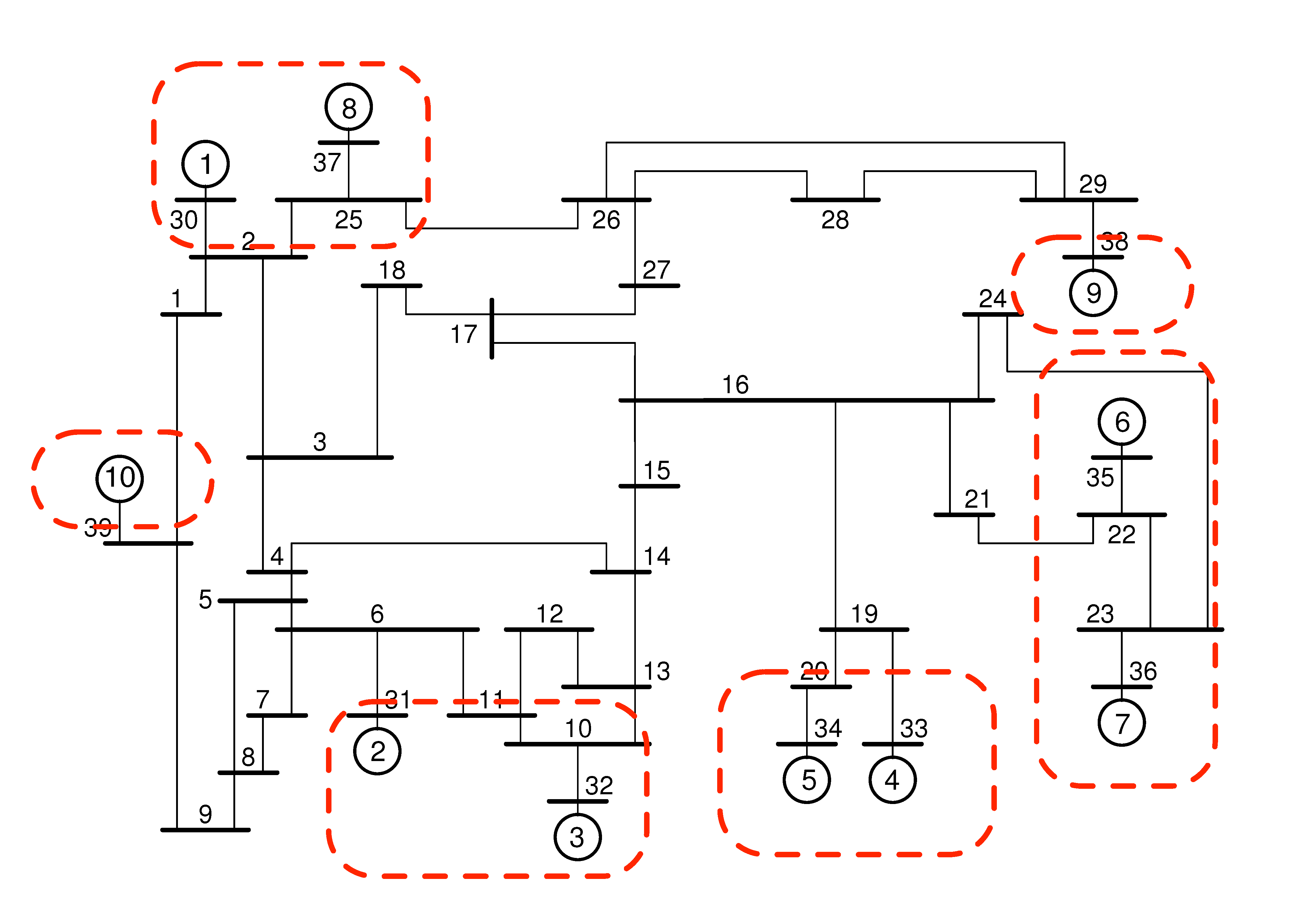Contributed by Florian Dorfler and Mihailo R. Jovanovic Matlab Files neWAC.m  —  A Matlab function required to run lqrsp.m. neData.mat  —  Data for the New England Power Grid example. neDataPST.m  —  Power System Toolbox data file. Presentations LANL Talk 2013 ACC Talk

## Problem description

The IEEE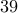New England Power Grid Model consists ofbuses and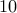generators. Generatoris an equivalent aggregated model for the part of the network that we do not have control over; generatorsto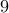are equipped with Power System Stabilizers (PSSs), which provide good damping of local modes and stabilize otherwise unstable open-loop system. We use a sparsity-promoting optimal control strategy to design a supplementary wide area control signal aimed at achieving a desirable trade-off between damping of the inter-area oscillations and communication requirements in the distributed controller. Inter-area oscillations are associated with the dynamics of power transfers and they are characterized by groups of coherent machines that swing relative to each other. These oscillations are caused by weakly damped modes of the linearized swing equations and they physically correspond to active power transfer between different generator groups.

In the absence of higher-order generator dynamics, for purely inductive lines and constant-current loads, the dynamics of each generator can be represented by the electromechanical swing equations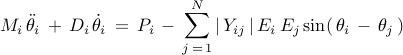where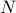denotes the number of generators,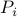is the generator power injection in the network-reduced model, and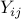is the Kron-reduced admittance matrix describing the interactions among generators. After linearization at an operating point the swing equations reduce to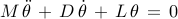where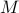and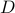are diagonal matrices of inertia and damping coefficients, and the coupling among generators is entirely described by the weighted graph induced by the Laplacian matrix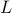.

Let the state of the power network be partitioned as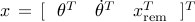where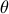and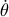are the rotor angles and frequencies ofsynchronous generators and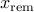are the state variables which correspond to fast electrical dynamics.

The sparsity-promoting minimum-variance optimal control problem can then be formulated as: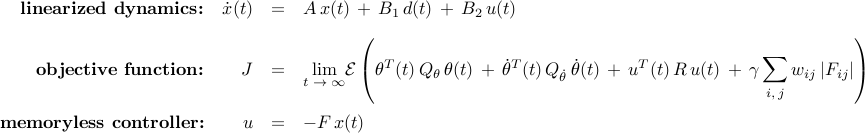where we use the weighted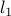norm to induce sparsity in the state feedback gain matrix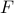.

## Computational results

We next present computations resulting from the use of the sparsity-promoting framework with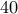logarithmically-spaced points for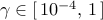. In the objective function we choose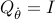,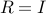, and set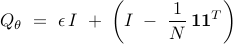in order to penalize deviation from synchrony. This choice of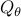is inspired by slow coherency theory with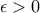denoting a small regularization parameter and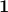denoting the vector of all ones.

Download Matlab code neWAC.m and data file neData.mat to reproduce these figures.

### Performance vs Sparsity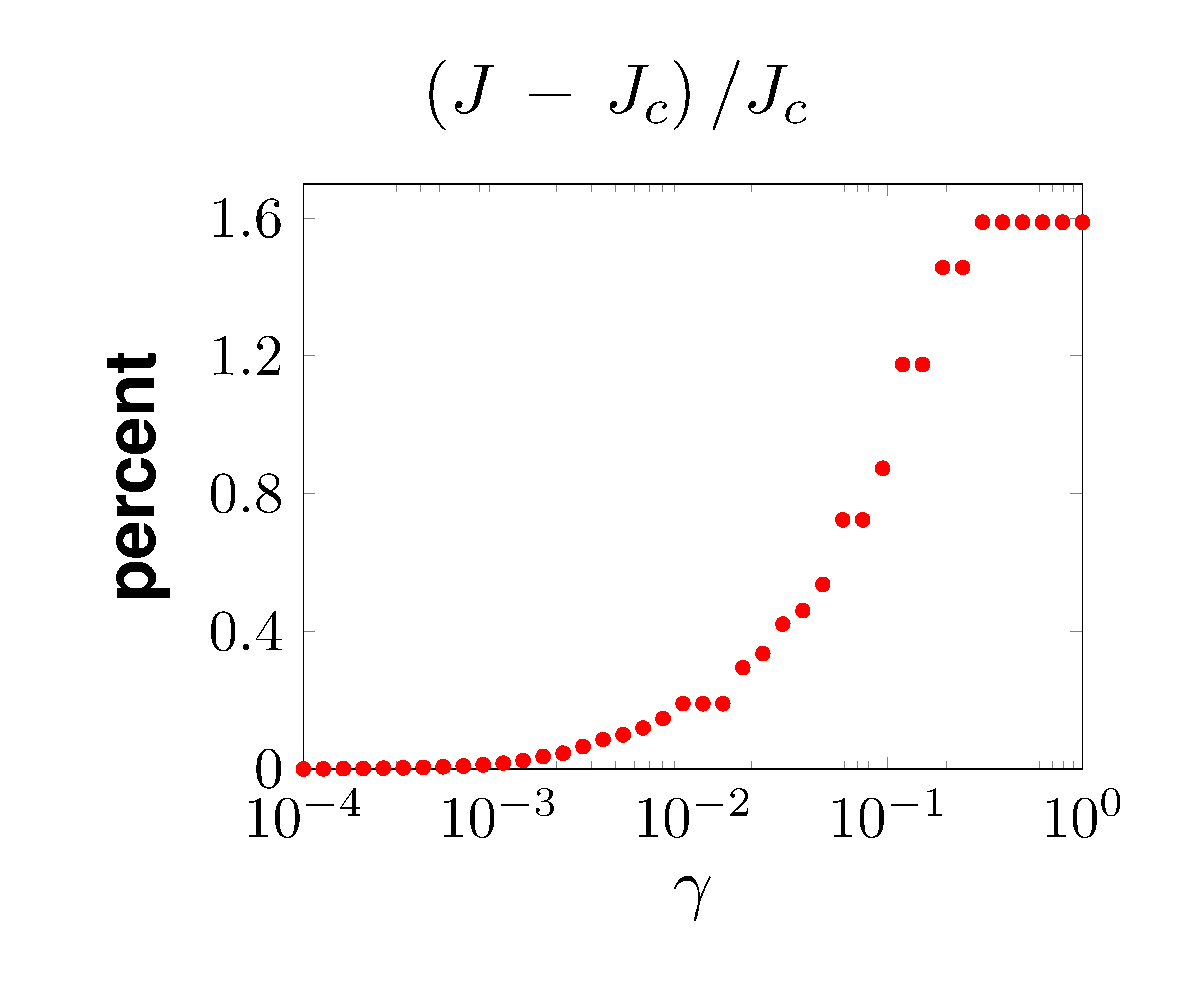Relative to the optimal centralized controller, performance of the optimal sparse controller deteriorates gracefully with increased emphasis on sparsity.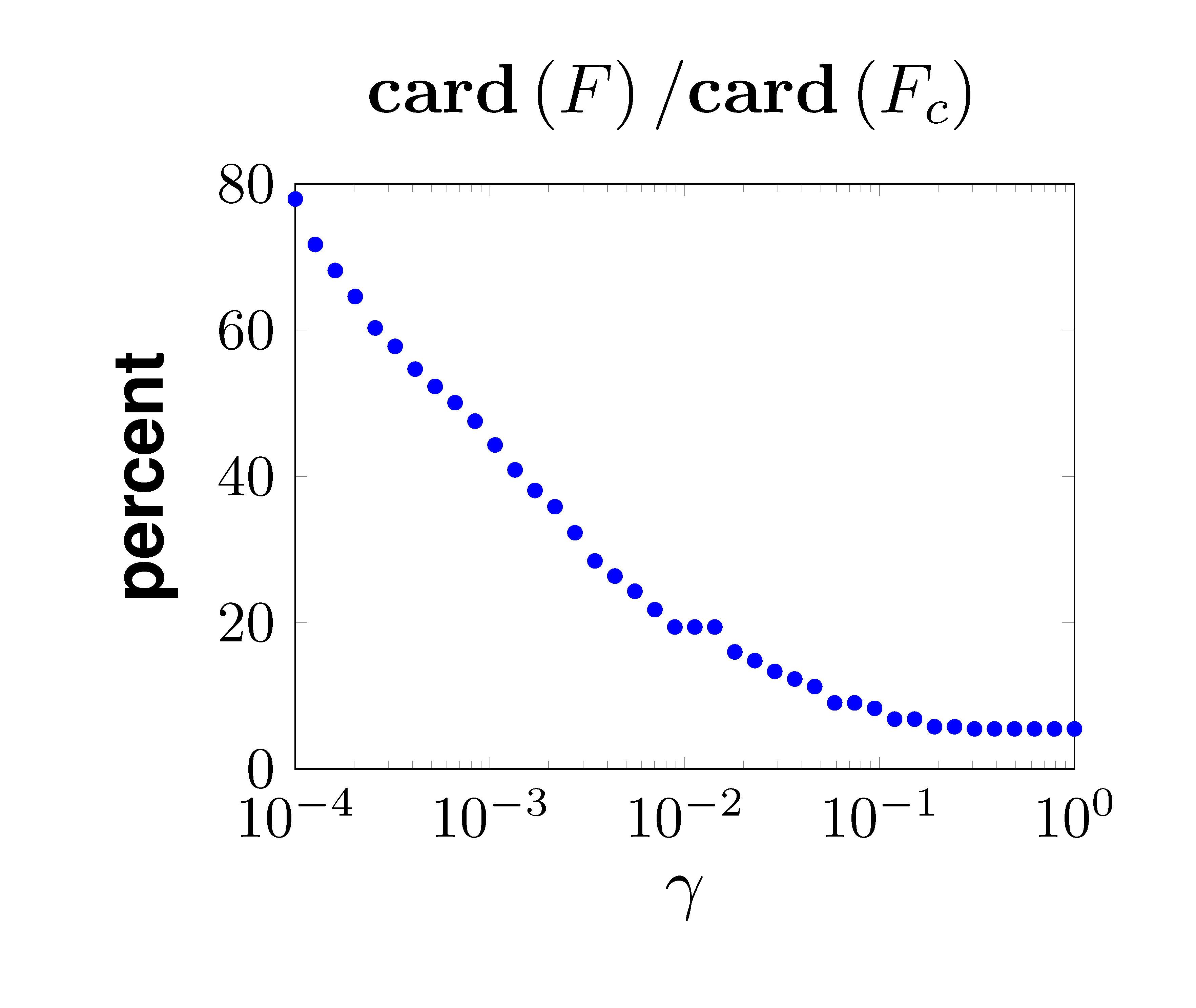For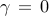, the optimal centralized feedback gain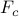is a dense matrix populated with nonzero elements. Increased emphasis on sparsity induces feedback gains with smaller number of nonzero elements.

### Signal exchange network

The sparsity pattern of the optimal feedback gain illustrates interactions between different generators. In the figures below, nine rows correspond to nine control inputs to controlled generators; the columns correspond to different states variables. Blue dots in each block represent local interactions within each generator, and red dots represent interactions between different generators.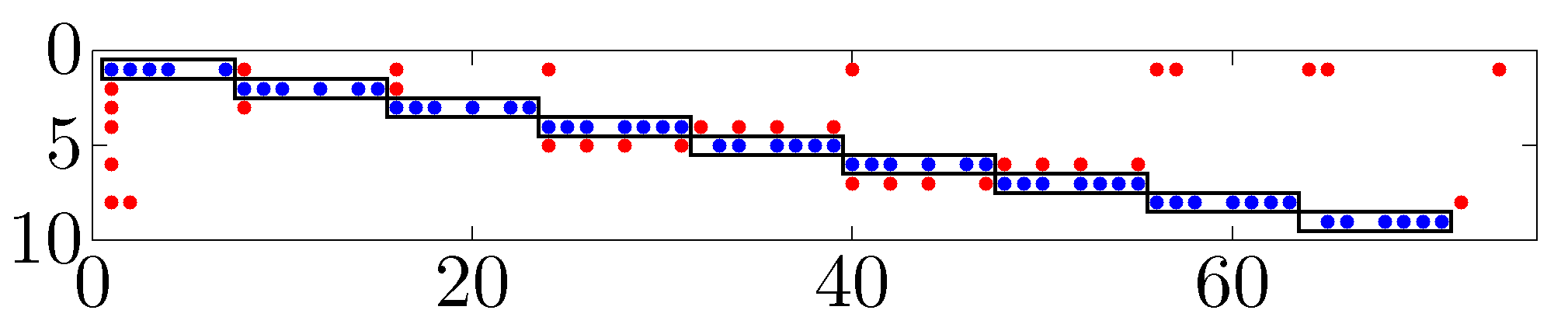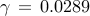,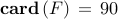Identified long range interactions are represented by red dots. The optimal sparse controller promotes the use of angles and frequencies (i.e., the first and second states of each subsystem) as signals to be communicated.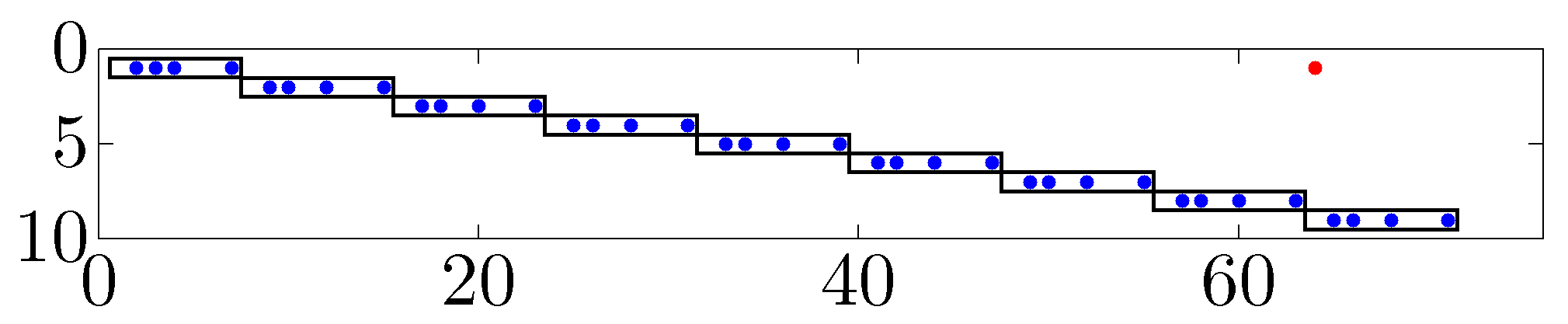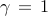,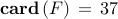Only a single long range interaction is identified: the controller at generatorneeds to access the angle of generator,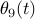. Relative to the optimal centralized feedback gain, the resulting sparse controller degrades performance by only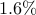.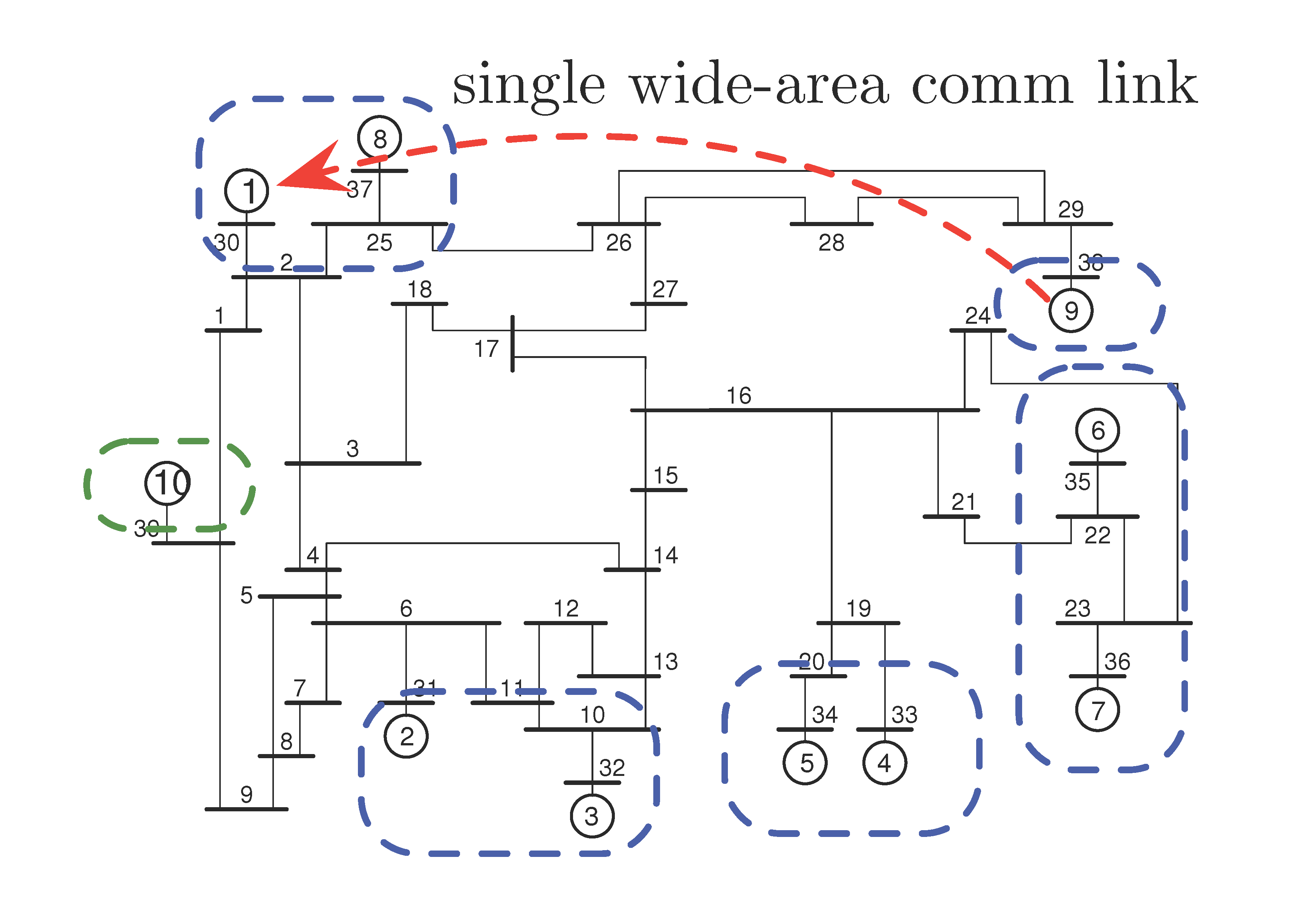As our emphasis on sparsity increases, most of the control burden is on generator, which has the largest inertia of all controlled generators. For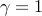, only the angle of loosely connected generatorneeds to be measured and communicated to generator.

### Dominant inter-area modes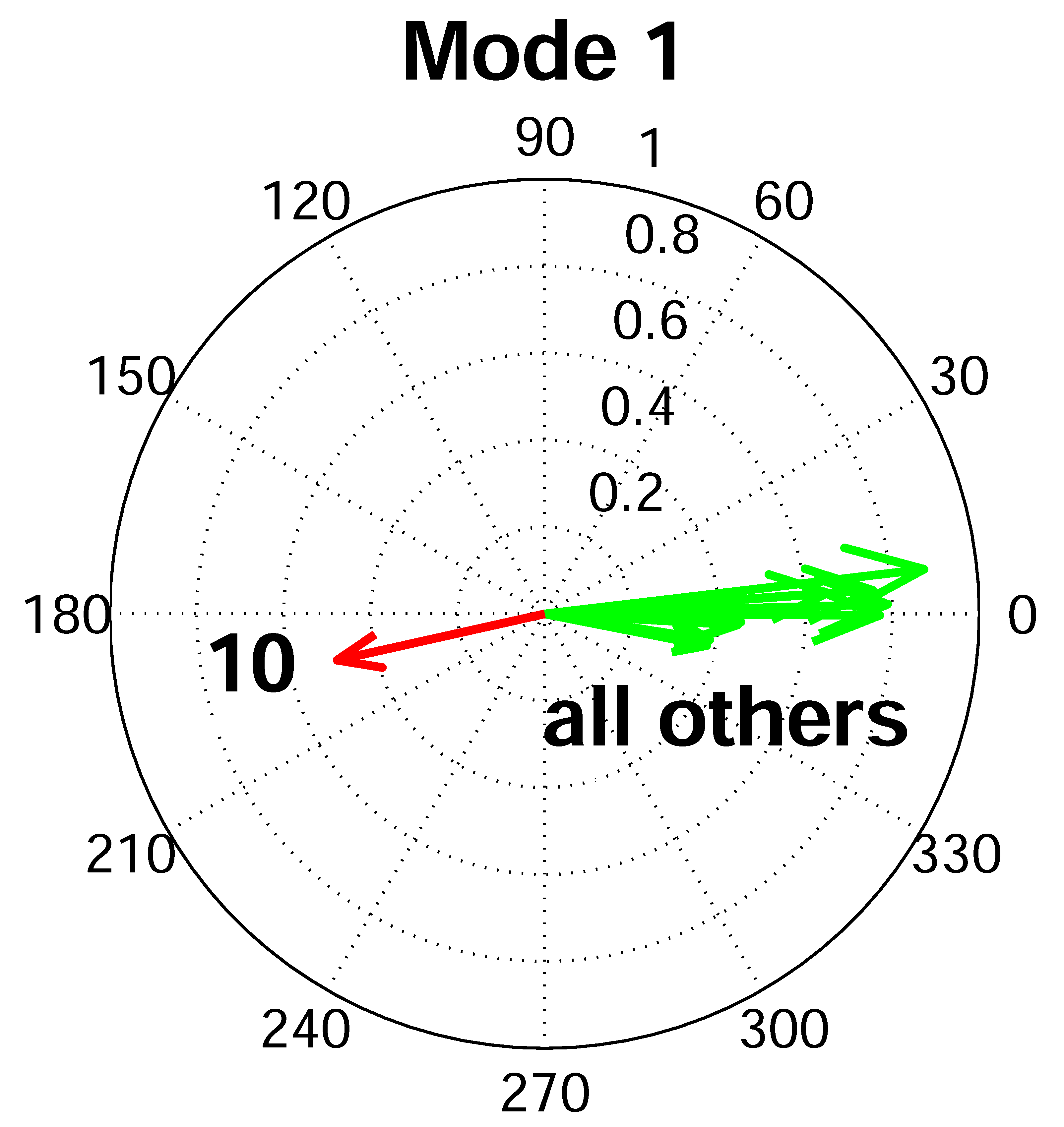The polar plots show the generator frequency components of one of the poorly damped inter-area modes in the open-loop system (i.e., the system controlled with only local PSSs). This inter-area mode is characterized by active power transfer between generatorand the rest of the grid.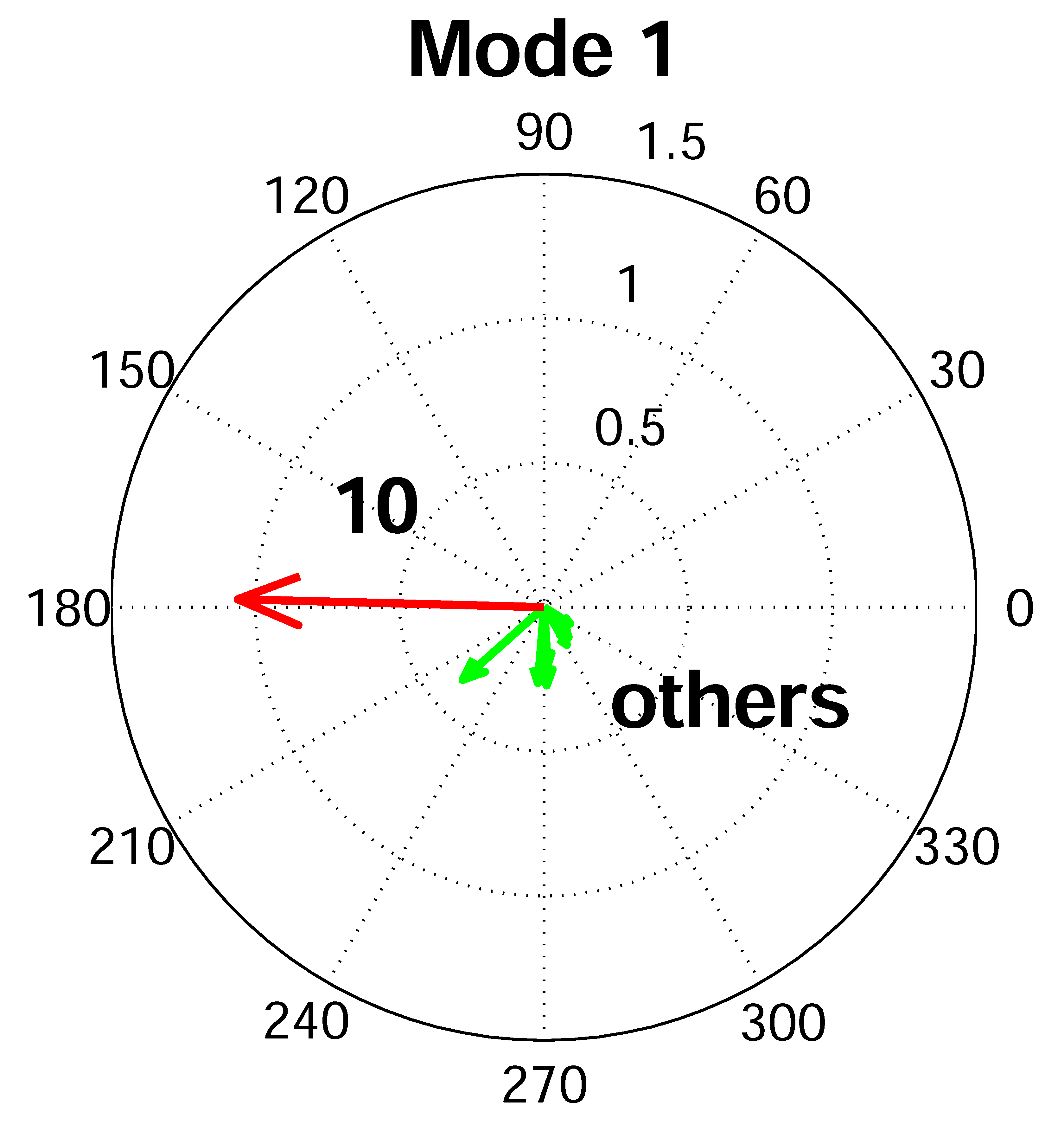The least damped inter-area mode of the closed-loop system (i.e., the system controlled with both local PSSs and the optimal sparse wide-area controller obtained for). Relative to the open-loop system, the closed-loop system is characterized by much smaller active power transfer between generatorand the rest of the grid.

## Citation

If you are using information provided here in your research or teaching, please include explicit mention of this website

www.umn.edu/~mihailo/software/lqrsp/

and of our papers.

```@article{dorjovchebulTPS14,
author  = {F. D\"orfler and M. R. Jovanovi\'c and M. Chertkov and F. Bullo},
title   = {Sparsity-promoting optimal wide-area control of power networks},
journal = {IEEE Trans. Power Syst.},
volume  = {29},
number  = {5},
pages   = {2281-2291},
year    = {2014}
}

@inproceedings{dorjovchebulACC13,
author       = {F. D\"orfler and M. R. Jovanovi\'c and M. Chertkov and F. Bullo},
booktitle    = {Proceedings of the 2013 American Control Conference},
title        = {Sparse and optimal wide-area damping control in power networks},
year         = {2013},